Sale!

# Deep Learning Online Course

\$149.00

Limited Time Offer

This course is for anyone who wants to learn about deep learning. No prior experience is necessary, although some knowledge of machine learning would be beneficial. This course is also suitable for anyone who wants to refresh their knowledge of deep learning.

## Deep Learning Online Course Overview:

In this course, you will learn the basics of deep learning. Upon completing this course, you will be able to build neural networks and understand how they work. You will also be able to apply your knowledge to real-world problems, such as image classification and natural language processing.

This course is divided into four weeks, each week focusing on a different aspect of deep learning. Week one will focus on the basics of neural networks, week two will focus on training neural networks, week three will focus on modern applications of neural networks, and week four will focus on advanced topics in deep learning.

By the end of this course, you will be able to:

– Understand the basics of neural networks

– Train a neural network

– Apply neural networks to real-world problems

– Understand advanced topics in deep learning

This course is for anyone who wants to learn about deep learning. No prior experience is necessary, although some knowledge of machine learning would be beneficial. This course is also suitable for anyone who wants to refresh their knowledge of deep learning.

### Curriculum:

Week 1:

– Introduction to Deep Learning

– What is a Neural Network?

– The Structure of a Neural Network

– Forward Propagation

– Activation Functions

Week 2:

– Training a Neural Network

– Backpropagation

– Optimization Algorithms

Week 3:

– Modern Applications of Neural Networks

– Computer Vision

– Natural Language Processing

Week 4:

– Advanced Topics in Deep Learning

– Generative Models

– Reinforcement Learning

Length: 4 weeks

Certificate Available: Yes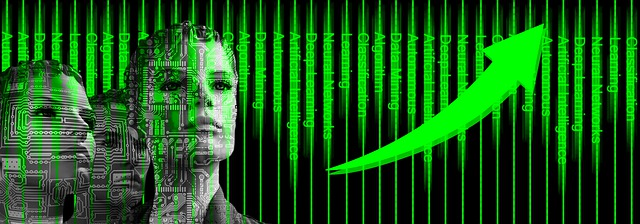## Sneak Peaks / Summary / Glossary

### What is Deep Learning?

Tell us the basic principles behind machine learning. Machine learning involves letting machines learn data entirely independently. Depending how you do this, it is possible to build machine learning algorithms that distinguish images of animals from photographs of animals.

At first, this algorithm may lack the ability to do that properly. However if we teach algorithms to differentiate cats and dogs, it will become more efficient.

Because learning is an indication of intelligence, machines can therefore become an integral component of artificial intelligence. Deep Learning combines machine learning with artificial neural networks to make predictions.

Deep learning is a subset of machine learning in artificial intelligence that has algorithms inspired by the structure and function of the brain called artificial neural networks.

Deep learning is a powerful tool that can be used for many different applications, such as image recognition, natural language processing, and time series prediction. In this course, we will focus on image recognition.

Image recognition is the process of identifying and classifying objects in digital images. There are many different approaches to image recognition, but deep learning is one of the most accurate and widely used methods.

Deep learning algorithms learn by example. They are given a dataset of images that have been labeled with the correct object classification, and they learn to identify the features that distinguish the different classes of objects.

For example, a deep learning algorithm might be given a dataset of images that contain dogs, cats, and rabbits. The algorithm will learn to identify the features that distinguish dogs from cats and rabbits.

The process of training a deep learning algorithm is called deep learning training.

Deep learning training is a process of optimizing a deep learning algorithm so that it can accurately make predictions on new data.

The process of training a deep learning algorithm involves adjusting the algorithms parameters, such as the weights and biases, to minimize prediction error.

Prediction error is the difference between the labels that the algorithm predicts and the actual labels of the data.

Deep learning training is a computationally intensive process, and it can take days or even weeks to train a deep learning algorithm on a large dataset.

Deep learning is a powerful tool for image recognition, but it is not perfect. Deep learning algorithms are often biased towards the class of objects that they are trained on.

For example, a deep learning algorithm that is trained on a dataset of images that contain only dogs will be biased towards dogs.

This means that the algorithm will be less accurate at recognizing other objects, such as cats and rabbits.

To reduce bias, it is important to train deep learning algorithms on a dataset that is as diverse as possible.

Diversity can be achieved by increasing the number of classes of objects, or by increasing the number of examples for each class.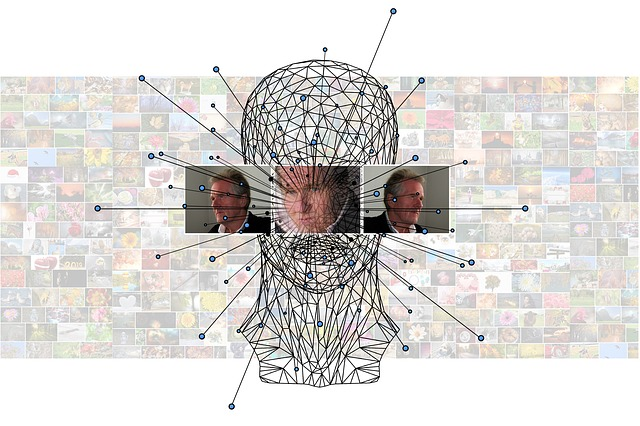### Is deep learning possible in online learning?

Havard et. al. also found that on-line learning could support critical thinkers deep learning because it provides a learning-centered environment.

In this environment, learners can take initiative and be engaged and responsible for their own learning.

### What are some of the benefits of deep learning?

Some benefits of deep learning include real-time image recognition, better understanding of how the brain works, facial recognition, and cancer detection. Additionally, deep learning can help with fraud detection, text analysis, and time series prediction.

### What is the best online course to learn deep learning?

The best online courses to learn deep learning depend on your level of expertise and experience. If you are a beginner, consider taking a course that covers the basics of machine learning and artificial intelligence. For those with more experience, there are plenty of advanced courses available.

### What are some of the challenges of deep learning?

Some challenges of deep learning include the need for large amounts of data, the lack of clarity on how to interpret results, and the potential for overfitting. Additionally, deep learning can be computationally intensive, so it is important to have access to powerful hardware. Finally, there is also the challenge of keeping up with the latest research as the field is constantly evolving.### Glossary of Terms and Concepts from the Course:

#### Deep learning:

A subfield of machine learning that is concerned with algorithms that learn from data that is too complex for humans to understand.

#### Training:

The process of optimizing a deep learning algorithm so that it can accurately make predictions on new data.

#### Prediction error:

The difference between the labels that the algorithm predicts and the actual labels of the data.

#### Machine learning:

A field of artificial intelligence that is concerned with algorithms that learn from data.

#### Artificial intelligence:

A field of computer science that is concerned with the development of algorithms that can make decisions for themselves.

#### Bias:

A tendency to prefer one thing over another. In machine learning, bias refers to the tendency of an algorithm to be inaccurate when dealing with new data that is different from the data that it was trained on.

#### Deep neural networks:

A type of deep learning algorithm that is inspired by the structure of the brain. Deep neural networks are composed of many layers of interconnected nodes, or neurons.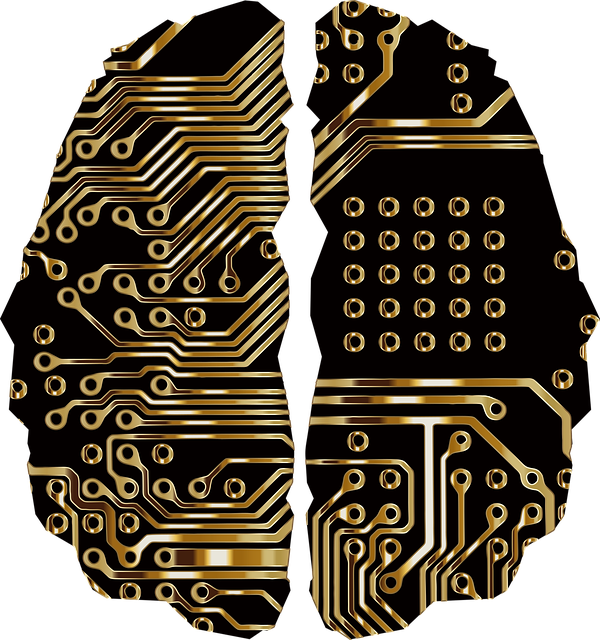#### Supervised learning:

A type of machine learning in which the algorithms are given labels along with the data so that they can learn to predict the label for new data.

#### Unsupervised learning:

A type of machine learning in which the algorithms are not given any labels and must learn to find patterns in the data on their own.

#### Overfitting:

The tendency of a machine learning algorithm to perform well on the data that it was trained on, but to generalize poorly to new data. Overfitting occurs when an algorithm has learned the noise in the data instead of the actual signal.

Regularization: A method of preventing overfitting by adding a term to the error function that penalizes complex models.

#### Dropout:

A regularization technique for deep neural networks in which nodes are randomly removed from the network during training. Dropout helps to prevent overfitting and improve the generalizability of the model.

#### Feature engineering:

The process of creating new features from existing data. Feature engineering is often used in machine learning to improve the performance of algorithms.

#### Convolutional neural networks:

A type of deep neural network that is particularly well suited for image recognition tasks. Convolutional neural networks are composed of layers of interconnected nodes, or neurons, that have been designed to mimic the way that the brain processes visual information.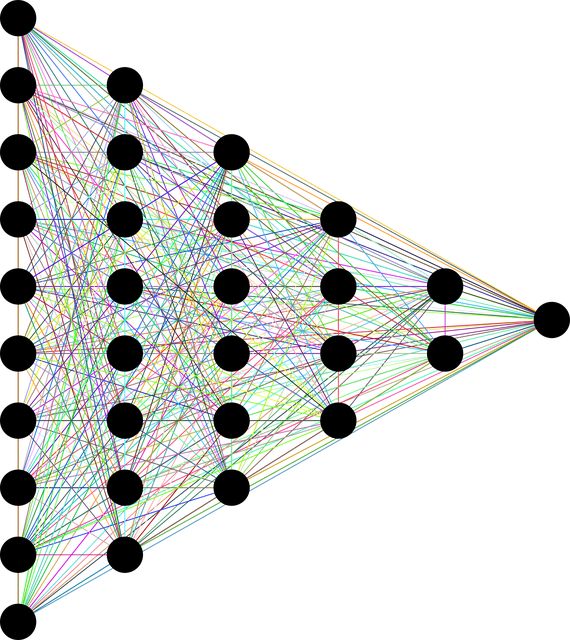#### Recurrent neural networks:

A type of deep neural network that is well suited for tasks that involve sequences of data, such as text or time series data. Recurrent neural networks are composed of layers of interconnected nodes, or neurons, that have been designed to allow information to flow through the network in a loop.

#### LSTM:

A type of recurrent neural network that is designed to overcome the vanishing gradient problem. LSTMs are composed of a series of interconnected cells that can remember information for long periods of time.

The tendency of the error function to plateau during training of deep neural networks. The vanishing gradient problem occurs because the gradients of the error function become smaller and smaller as they flow back through the network.

#### Reinforcement learning:

A type of machine learning in which algorithms learn by trial and error. Reinforcement learning is often used in robotics, where an algorithm can learn to control a robot by trial and error.

#### Q-learning:

A type of reinforcement learning in which an algorithm learns by approximating the value function. Q-learning is often used in robotics, where an algorithm can learn to control a robot by approximating the value of each state.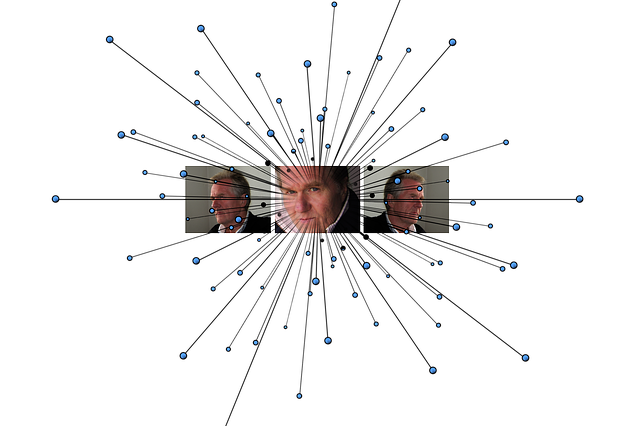#### Exploration vs. exploitation:

The exploration-exploitation tradeoff is a common challenge in machine learning. On the one hand, algorithms need to explore the data in order to find patterns and learn from them. On the other hand, they need to exploit the knowledge they have acquired in order to make accurate predictions. The balance between exploration and exploitation is often a delicate one, and it can be difficult to find the right balance.

#### Deep learning models:

Deep learning is a branch of machine learning that is concerned with the design of algorithms that can learn from data that is too complex for traditional machine learning methods. Deep learning models are composed of many layers of interconnected nodes, or neurons, and they are designed to mimic the way that the brain processes information. Deep learning models have been shown to be effective for a variety of tasks, including image recognition, natural language processing, and machine translation.

#### Transfer learning:

Transfer learning is a method of machine learning that allows knowledge to be transferred from one task to another. Transfer learning is often used when there is a limited amount of data available for the target task. By using knowledge from a source task, a model can learn to perform the target task with less data.

#### Continuous learning:

Continuous learning is a type of machine learning that allows models to continue to learn and improve as new data is collected. Continuous learning is often used in applications where data is constantly being generated, such as in sensors or monitoring systems.

#### Deep learning techniques:

Deep learning is a branch of machine learning that is concerned with the design of algorithms that can learn from data that is too complex for traditional machine learning methods. Deep learning models are composed of many layers of interconnected nodes, or neurons, and they are designed to mimic the way that the brain processes information. Deep learning models have been shown to be effective for a variety of tasks, including image recognition, natural language processing, and machine translation. Some common deep learning techniques include convolutional neural networks, recurrent neural networks, and deep belief networks.

#### Machine learning models:

A machine learning model is a mathematical model that is used to learn from data. Machine learning models are often used in applications where it is difficult or impossible for humans to write the rules for making predictions. Some common machine learning models include linear regression, support vector machines, and decision trees.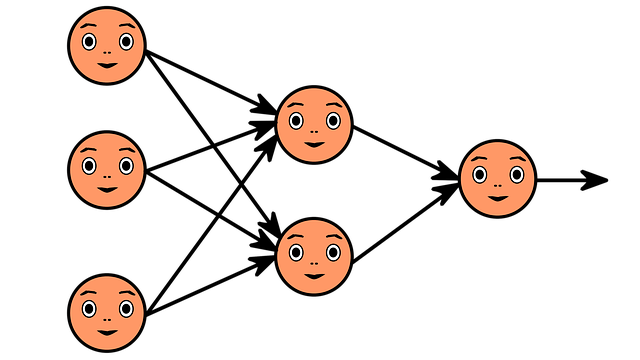#### Machine learning projects:

A machine learning project is a task or problem that can be solved using machine learning. Machine learning projects often involve large datasets and require the use of specialized hardware. Some common machine learning projects include image classification, facial recognition, and Recommender Systems.

#### Supervised learning:

Supervised learning is a type of machine learning in which algorithms learn from labeled training data. Supervised learning is often used in applications where there is a known set of correct answers, such as in image classification or spam detection.

#### Unsupervised learning:

Unsupervised learning is a type of machine learning in which algorithms learn from unlabeled data. Unsupervised learning is often used in applications where the

#### Machine Learning Engineer:

A machine learning engineer is a person who designs and builds machine learning models. Machine learning engineers often have a background in computer science or engineering.

#### Data Scientist:

A data scientist is a person who extracts insights from data. Data scientists often have a background in statistics or math.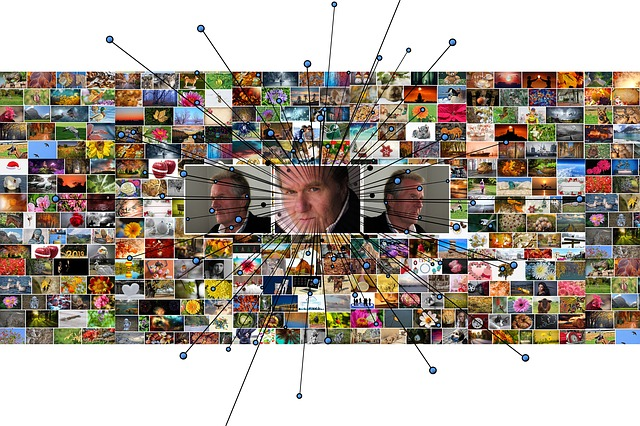#### Computer vision:

Computer vision is the field of AI that deals with how computers can be made to understand digital images. Computer vision is often used in applications such as image classification, object detection, and facial recognition.

#### Natural language processing:

Natural language processing is the field of AI that deals with how computers can be made to understand human language. Natural language processing is often used in applications such as machine translation, text classification, and sentiment analysis.

#### Deep learning skills:

Deep learning is a branch of machine learning that is concerned with the design of algorithms that can learn from data that is too complex for traditional machine learning methods. Deep learning models are composed of many layers of interconnected nodes, or neurons, and they are designed to mimic the way that the brain processes information. Deep learning models have been shown to be effective for a variety of tasks, including image recognition, natural language processing, and machine translation. Some common deep learning techniques include convolutional neural networks, recurrent neural networks, and deep belief networks.

#### Speech recognition:

Speech recognition is the process of converting spoken words into text. Speech recognition is often used in applications such as voice search and automatic captioning.

#### Predictive modeling:

Predictive modeling is the process of using machine learning to make predictions about future events. Predictive modeling is often used in applications such as weather forecasting, stock market prediction, and fraud detection.#### Recommender systems:

Recommender systems are a type of artificial intelligence that are used to predict what products or services a user might be interested in.Recommender systems are often used in applications such as e-commerce and social media.

#### Anomaly detection:

Anomaly detection is the process of identifying data points that are unusual or unexpected.

#### Reinforcement learning:

Reinforcement learning is a type of machine learning in which algorithms learn by trial and error. Reinforcement learning is often used in applications such as games, robotics, and autonomous vehicles.

Debuggi: Debugging is the process of identifying and fixing errors in software. Debugging is often done by developers during the software development process.

#### Profiling:

Profiling is the process of measuring the performance of a software program. Profiling is often used to find bottlenecks in code.

#### Optimization:

Optimization is the process of making a system or process more efficient. Optimization is often used in applications such as resource planning and asset management.

#### Computer science:

Computer science is the study of the principles and applications of computing. Computer science is often used in applications such as software engineering, artificial intelligence, and computer graphics.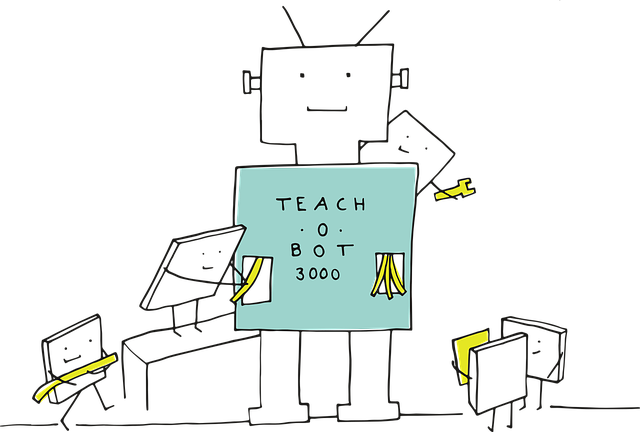#### Algorithms:

An algorithm is a set of instructions that are followed in order to solve a problem. Algorithms are often used in applications such as data mining and machine learning.

#### Linear algebra:

Linear algebra is the branch of mathematics that deals with the study of lines and planes. Linear algebra is often used in applications such as physics and engineering.

#### Calculus:

Calculus is the branch of mathematics that deals with the study of change. Calculus is often used in applications such as physics and engineering.

#### Statistics:

Statistics is the branch of mathematics that deals with the collection, analysis, and interpretation of data. Statistics is often used in applications such as data mining and machine learning.

#### Data structures:

A data structure is a way of organizing data so that it can be efficiently accessed and manipulated. Data structures are often used in applications such as databases and computer networks.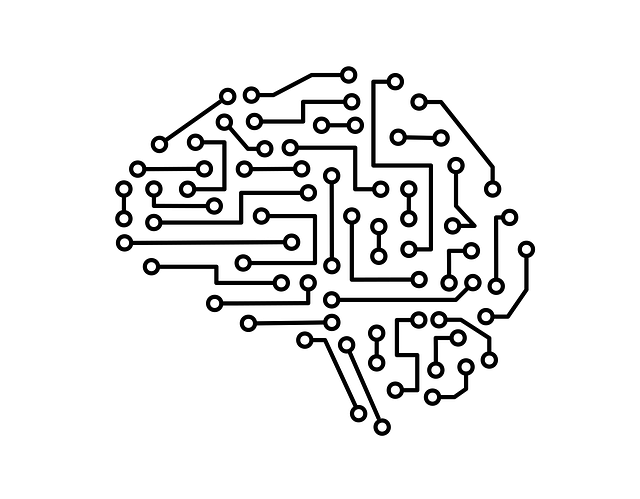#### Discrete mathematics:

Discrete mathematics is the branch of mathematics that deals with the study of discrete objects. Discrete mathematics is often used in applications such as computer science and engineering.

#### Combinatorics:

Combinatorics is the branch of mathematics that deals with the study of combinations. Combinatorics is often used in applications such as data mining and machine learning.

#### Number theory:

Number theory is the branch of mathematics that deals with the study of numbers. Number theory is often used in applications such as cryptography and data compression.

#### Functional programming:

Functional programming is a style of programming that emphasizes the use of functions rather than objects. Functional programming is often used in applications such as image processing and scientific computing.

#### Logic:

Logic is the branch of mathematics that deals with the study of deduction. Logic is often used in applications such as computer science and engineering.

#### Set theory:

Set theory is the branch of mathematics that deals with the study of sets. Set theory is often used in applications such as data mining and machine learning.

#### Probability:

Probability is the branch of mathematics that deals with the study of chance. Probability is often used in applications such as statistics and data mining.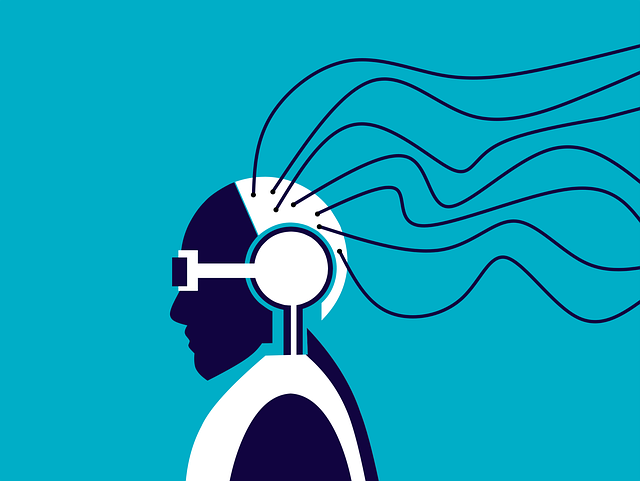#### Neural network’s architecture:

Neural networks are a type of machine learning algorithm that are used to model complex patterns in data. Neural networks are often used in applications such as image recognition and classification.

#### Neural network theory:

Neural network theory is the branch of mathematics that deals with the study of neural networks. Neural network theory is often used in applications such as computer science and engineering.

#### Continuous optimization:

Continuous optimization is the process of finding the optimum value of a function by iteratively improving the estimates of the function’s gradient. Continuous optimization is often used in applications such as machine learning and engineering.

#### Convex optimization:

Convex optimization is a type of optimization problem where the objective function is convex. Convex optimization is often used in applications such as machine learning and engineering.

#### Nonlinear optimization:

Nonlinear optimization is the process of finding the optimum value of a function by iteratively improving the estimates of the function’s gradient. Nonlinear optimization is often used in applications such as machine learning and engineering.

#### Integer programming:

Integer programming is a type of optimization problem where the variables are constrained to be integers. Integer programming is often used in applications such as operations research and engineering.

#### Linear programming:

Linear programming is a type of optimization problem where the objective function is linear. Linear programming is often used in applications such as operations research and engineering.

Quadratic programming is a type of optimization problem where the objective function is quadratic. Quadratic programming is often used in applications such as machine learning and engineering.

#### Calculus:

Calculus is the branch of mathematics that deals with the study of change. Calculus is often used in applications such as physics and engineering.

#### Differential equations:

Differential equations are a type of equation that describes how a function changes over time. Differential equations are often used in applications such as physics and engineering.

#### Linear algebra:

Linear algebra is the branch of mathematics that deals with the study of vectors and matrices. Linear algebra is often used in applications such as computer science and engineering.

#### Discrete mathematics:

Discrete mathematics is the branch of mathematics that deals with the study of finite objects. Discrete mathematics is often used in applications such as computer science and engineering.#### Computational geometry:

Computational geometry is the branch of mathematics that deals with the study of algorithms for manipulating geometric objects. Computational geometry is often used in applications such as computer graphics and engineering.

Number theory:

Number theory is the branch of mathematics that deals with the study of integers. Number theory is often used in applications such as cryptography and engineering.

#### Algebra:

Algebra is the branch of mathematics that deals with the study of equations and variables. Algebra is often used in applications such as physics and engineering.

#### Geometry:

Geometry is the branch of mathematics that deals with the study of shapes and sizes. Geometry is often used in applications such as architecture and engineering.

#### Trigonometry:

Trigonometry is the branch of mathematics that deals with the study of triangles. Trigonometry is often used in applications such as physics and engineering.

#### Statistics:

Statistics is the branch of mathematics that deals with the collection, analysis, and interpretation of data. Statistics is often used in applications such as market research and engineering.

#### Probability:

Probability is the branch of mathematics that deals with the study of chance. Probability is often used in applications such as statistics and engineering.

There is no doubt that AI technology is developing at a rapid pace. New applications and use cases for AI are being discovered all the time, and the technology is becoming more sophisticated and powerful. As such, it is important to stay up-to-date with the latest AI technology so that you can make use of it in your own applications.

One of the most common use cases for AI is language reading. This involves using AI to read and understand text in a given language. This can be used for a variety of tasks such as machine translation, document processing, and natural language understanding.

#### NLP:

NLP is a branch of AI that deals with the process of understanding and generating human language. NLP is used for tasks such as machine translation, text summarization, and question answering.

#### Speech recognition:

Speech recognition is the process of converting spoken words into text. This can be used for tasks such as voice search and automatic transcription.#### Sentiment analysis:

Sentiment analysis is the process of understanding the emotions expressed in text. This can be used for tasks such as customer service and marketing research.

#### Predictive analytics:

Predictive analytics is the process of using data to make predictions about future events. This can be used for tasks such as fraud detection and product recommendations.